International
Tables for
Crystallography
Volume H
Powder diffraction
Edited by C. J. Gilmore, J. A. Kaduk and H. Schenk

International Tables for Crystallography (2018). Vol. H, ch. 3.6, pp. 288-289

## Section 3.6.1. Introduction

M. Leonia*

aDepartment of Civil, Environmental and Mechanical Engineering, University of Trento, via Mesiano 77, 38123 Trento, Italy
Correspondence e-mail: Matteo.Leoni@unitn.it

### 3.6.1. Introduction

| top | pdf |

X-ray diffraction is a very simple technique, but is one of the most flexible and powerful tools for the analysis of materials. The diffraction pattern carries information about the atomic arrangement and motion at both the short and the long range; for nanostructured materials this means that a single technique can simultaneously provide structural and microstructural information.

Microstructure analysis via X-ray powder diffraction (XRD), often termed line-profile analysis (LPA), is mostly performed through the Scherrer (1918) formula. Just a few years after the discovery of X-ray diffraction, Scherrer derived a very simple relationship between the width of the diffraction peaks and the size of the so-called Kristallchen (translated as crystallites), the coherently scattering (nanocrystalline) domains composing the colloids that he was studying (the formula is rewritten here using an updated notation):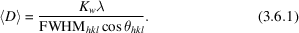The calculation of an average size' <D> is therefore immediate once the position and full-width at half-maximum of a peak (2θhkl and FWHMhkl, respectively), measured with X-rays of wavelength λ, are available. The constant Kw (the Scherrer constant) carries information on the shape of the domains and has an order of magnitude of 1. Values of the Scherrer constant can be found in the literature for both isotropic and anisotropic shapes (in the latter case leading to different sizes for different reflections hkl): Table 3.6.1contains the data of Langford & Wilson (1978) for common domain shapes. An elegant derivation of the Scherrer formula can be found in the work of Patterson (1939) and Warren (1990); a summary is also presented in Chapter 5.1.

 Table 3.6.1| top | pdf | Scherrer constants (Kw and Kβ) for various domain shapes (Langford & Wilson, 1978)
ShapeKw (FWHM)Kβ (integral breadth)
Sphere 0.89 1.07
Cube 0.83–0.91 1.00–1.16
Tetrahedron 0.73–1.03 0.94–1.39
Octahedron 0.82–0.94 1.04–1.14

Its simple mathematical nature is probably the main reason for the widespread (ab)use of equation (3.6.1). Simple, in fact, does not mean accurate.

The Scherrer formula and its variants are based on strong assumptions about the peak shape. In the original derivation [equation (3.6.1)] the peak was assumed to be Gaussian (see Appendix A3.6.1for the definition of a unit-area Gaussian); in subsequent derivations, the peak-shape information is lost, as the peak is transformed into an equivalent rectangle via the use of the integral breadth (IB) β = A/I, where A and I are the area and the maximum intensity of the peak, respectively (see Table 3.6.1for the corresponding Scherrer constant values). Together with this, we should consider that the size of the domains in a real specimen is always disperse; it can be easily proven that the quantity <D>, which is called the average size' or mean size', is actually not the mean (first moment) of the size distribution, but is related to its third moment (i.e. it is volume-weighted). If we add that the finite size of the domains is not the only source of peak broadening, we immediately see where the abuse of the Scherrer formula can lie.

To try to sort some of those issues out, Williamson & Hall (1953) proposed plotting the FWHM (or the IB) versus the reciprocal of the lattice spacing (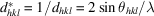). For spherical domains (i.e. size independent of the direction), a horizontal line is expected. An anisotropic shape would cause a scattering of the points, whereas other sources of broadening might also change the slope. Following the findings of Stokes & Wilson (1944), Williamson and Hall proposed writing the integral breadth in reciprocal space (reciprocal-space variable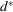) as a combination of the Scherrer formula with the differential of Bragg's law: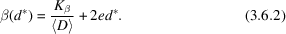Equation (3.6.2)describes a line for which the intercept (extrapolation of the integral breadth to the origin of the reciprocal space, i.e. to d→∞) is related to the reciprocal of the Scherrer size, and the slope parameter e accounts for the distribution of local strain inside the domains. For a Gaussian distribution of this local strain, the root-mean strain (also known as microstrain) <2>1/2 =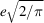can be obtained. The microstrain, which is mostly caused by the presence of imperfections, is often quoted together with the average size.

Even though the Williamson–Hall idea is straightforward, there is no physical reason why the two terms in equation (3.6.2)should be added: the only case where breadths are additive is when the peaks are Lorentzian (see Appendix A3.6.1for the definition of a unit-area Lorentzian). The Williamson–Hall equation is therefore valid for Lorentzian peaks and under the condition that both the size and strain contributions are Lorentzian as well. We therefore immediately envisage a problem here, as the size contribution, described by the Scherrer equation, was derived in the Gaussian limit. This inconsistency is seldom reported or considered in the literature. The fact that, in the end, the profiles are often highly Lorentzian in character mathematically justifies the separation of a size and a strain term, but dilutes the quantitative meaning of the result.

Modification of the Williamson–Hall approach to remove the inconsistency of the size- and strain-broadening terms has been extensively discussed by Balzar & Popović (1996). Using Voigt­ians (i.e. the convolution of a Gaussian G and a Lorentzian L; see Appendix A3.6.1) to describe a profile, four combinations are possible for the size and strain terms: LL, LG, GL and GG. The Williamson–Hall method corresponds to the LL case, whereas the combinations involving a Gaussian size term are more compatible with the Scherrer formula. Even in those cases, though, The pure-Gauss size-broadened profile is incompatible with the definitions of surface-weighted domain size and column-length distribution function' (Balzar & Popović, 1996): integral breadth methods are therefore intrinsically limited for the microstructure analysis of real materials. This is in stark contrast to the fact that equation (3.6.2)is used, for example, by a large number of Rietveld refinement codes to describe the observed trend in line-profile broadening (and to perform a rough microstructure analysis). This situation can be improved a little by properly considering at least the anisotropic broadening component, as performed, for example, in the modified Williamson–Hall method (MWH; Ungár & Borbély, 1996; Ungár, 2001; Scardi et al., 2004). The results are more accurate and related to some physical quantities (a dislocation density and a stacking fault probability), but they are still tightly bound to a Voigtian profile approximation.

An alternative to the integral breadth methods was developed by Warren & Averbach (1950, 1952) almost simultaneously with the idea of Williamson and Hall, but took longer to be fully employed owing to the lack of fast computing tools. It is based on the extensive use of Fourier transforms and represents the starting point of modern line-profile analysis techniques. The whole profile carries information on the microstructure, as each point in reciprocal space is related to the Fourier transform of real space (and thus to the size and shape of the domains and also the deviation from perfect three-dimensional periodicity). Each profile also contributes to a better picture of the microstructure, as it samples along a different direction in space.

For decades, these Fourier methods were only used in a very small number of scientific areas; the availability of fast computers and the fast Fourier transform has contributed greatly to their further diffusion. It is, however, only in recent years that the full power of the Fourier approach has been unveiled, with the development of whole-pattern methods and the extension of most models to a wider range of materials.

### References

Balzar, D. & Popović, S. (1996). Reliability of the simplified integral-breadth methods in diffraction line-broadening analysis. J. Appl. Cryst. 29, 16–23.Google Scholar
Langford, J. I. & Wilson, A. J. C. (1978). Scherrer after sixty years: a survey and some new results in the determination of crystallite size. J. Appl. Cryst. 11, 102–113.Google Scholar
Patterson, A. L. (1939). The Scherrer formula for X-ray particle size determination. Phys. Rev. 56, 978–982.Google Scholar
Scardi, P., Leoni, M. & Delhez, R. (2004). Line broadening analysis using integral breadth methods: a critical review. J. Appl. Cryst. 37, 381–390.Google Scholar
Scherrer, P. (1918). Bestimmung der Grösse und der inneren Struktur von Kolloidteilchen mittels Röntgenstrahlen. Nachr. Ges. Wiss. Göttingen, pp. 98–100.Google Scholar
Stokes, A. R. & Wilson, A. J. C. (1944). The diffraction of X-rays by distorted crystal aggregates – I. Proc. Phys. Soc. 56, 174–181.Google Scholar
Ungár, T. (2001). Dislocation densities, arrangements and character from X-ray diffraction experiments. Mater. Sci. Eng. A Struct. Mater. 309–310, 14–22.Google Scholar
Ungár, T. & Borbély, A. (1996). The effect of dislocation contrast on X-ray line broadening: a new approach to line profile analysis. Appl. Phys. Lett. 69, 3173.Google Scholar
Warren, B. E. (1990). X-ray Diffraction. New York: Dover Publications. (Unabridged reprint of the original 1969 book.)Google Scholar
Warren, B. E. & Averbach, B. L. (1950). The effect of cold-work distortion on X-ray patterns. J. Appl. Phys. 21, 595–599.Google Scholar
Warren, B. E. & Averbach, B. L. (1952). The separation of cold-work distortion and particle size broadening in X-ray patterns. J. Appl. Phys. 23, 492.Google Scholar
Williamson, G. K. & Hall, W. H. (1953). X-ray line broadening from filed aluminium and wolfram. Acta Metall. 1, 22–31.Google Scholar